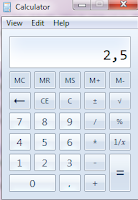## What is 5% of 50?

What is 5% of 50?
5% x 50 =
(5 : 100) x 50 =
(5 x 50 ) : 100 =
250 : 100  = 2,5

5% of 50 = 2,5Table example

5% of 50 = 2,5
10% of 50 = 5
15% of 50 = 7,5
20% of 50 = 10
25% of 50 = 12,5
30% of 50 = 15
35% of 50 = 17,5
40% of 50 = 20
45% of 50 = 22,5
50% of 50 = 25
55% of 50 = 27,5
60% of 50 = 30
65% of 50 = 32,5
70% of 50 = 35
75% of 50 = 37,5
80% of 50 = 40
85% of 50 = 42,5
90% of 50 = 45
95% of 50 = 47,5
100% of 50 = 50

1 . What is the percent of 5 out of 50?
5 : 50 = 0,1 = 10 %

2. What is 50 percent of 5? or what is 50% of 5 ?
Answer = 50 % of 5
50 : 100 x 5 = 2,5 or 2 1/2

3. What percent is 5 of 50?

4. How do you answer what is 5 percent of 50?
5% x 50 =
(5 : 100) x 50 =
(5 x 50 ) : 100 =
250 : 100  = 2,5